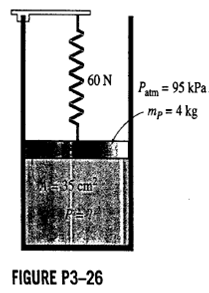×
Get Full Access to Fluid Mechanics - 2 Edition - Chapter 3 - Problem 23p
Get Full Access to Fluid Mechanics - 2 Edition - Chapter 3 - Problem 23p

×

# A gas is contained in a vertical, frictionlessISBN: 9780071284219 39

## Solution for problem 23P Chapter 3

Fluid Mechanics | 2nd Edition

• Textbook Solutions
• 2901 Step-by-step solutions solved by professors and subject experts
• Get 24/7 help from StudySoup virtual teaching assistantsFluid Mechanics | 2nd Edition

4 5 1 264 Reviews
22
1
Problem 23P

A gas is contained in a vertical, frictionless piston–cylinder device. The piston has a mass of 4 kg and a cross– sectional area of 35 $$\mathrm{Cm}^{2}$$. A compressed spring above the piston exerts a force of 60 N on the piston. If the atmospheric pressure is 95 kPa, determine the pressure inside the cylinder.Step-by-Step Solution:

Step 1 of 5

Mass of the piston m = 4 kg

Area of cross section of the piston A =orForce exerted by the spring F = 60 N

The atmospheric pressurekPa

Acceleration due to gravity g = 9.8_______________________

Step 2 of 5

Step 3 of 5

##### ISBN: 9780071284219

The full step-by-step solution to problem: 23P from chapter: 3 was answered by , our top Engineering and Tech solution expert on 07/03/17, 04:51AM. The answer to “?A gas is contained in a vertical, frictionless piston–cylinder device. The piston has a mass of 4 kg and a cross– sectional area of 35 $$\mathrm{Cm}^{2}$$. A compressed spring above the piston exerts a force of 60 N on the piston. If the atmospheric pressure is 95 kPa, determine the pressure inside the cylinder.” is broken down into a number of easy to follow steps, and 54 words. Since the solution to 23P from 3 chapter was answered, more than 3190 students have viewed the full step-by-step answer. This full solution covers the following key subjects: piston, pressure, cylinder, frictionless, Cross. This expansive textbook survival guide covers 15 chapters, and 1547 solutions. Fluid Mechanics was written by and is associated to the ISBN: 9780071284219. This textbook survival guide was created for the textbook: Fluid Mechanics, edition: 2.

## Discover and learn what students are asking

Calculus: Early Transcendental Functions : Antiderivatives and Indefinite Integration
?In Exercises 7–10, complete the table to find the indefinite integral. Original Integral Rewrite

Unlock Textbook Solution